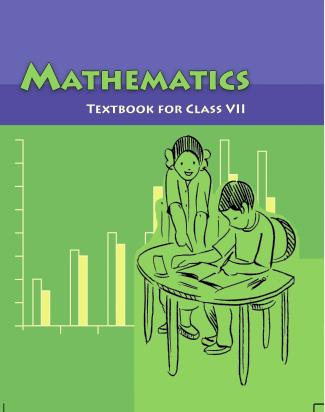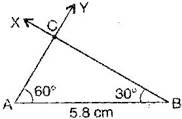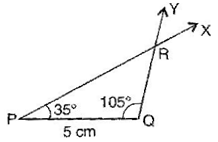1. /
2. CBSE
3. /
4. Class 07
5. /
6. Mathematics
7. /
8. NCERT Solutions for Class...

# NCERT Solutions for Class 7 Maths Exercise 10.4### myCBSEguide App

Download the app to get CBSE Sample Papers 2023-24, NCERT Solutions (Revised), Most Important Questions, Previous Year Question Bank, Mock Tests, and Detailed Notes.

NCERT solutions for Maths Practical Geometry## NCERT Solutions for Class 7 Maths Practical Geometry

###### Question 1.Construct {tex}\Delta {/tex}ABC, given {tex}m\angle {/tex}A = {tex}{60^ \circ },{/tex}{tex}m\angle {/tex}B = {tex}{30^ \circ }{/tex} and AB = 5.8 cm.

To construct: {tex}\Delta {/tex}ABC where {tex}m\angle {/tex}A = {tex}{60^ \circ },{/tex}{tex}m\angle {/tex}B = {tex}{30^ \circ }{/tex} and AB = 5.8 cm.Steps of construction:

(a) Draw a line segment AB = 5.8 cm.

(b) At point A, draw an angle {tex}\angle {/tex}YAB = {tex}{60^ \circ }{/tex} with the help of compass.

(c) At point B, draw {tex}\angle {/tex}XBA = {tex}{30^ \circ }{/tex} with the help of compass.

(d) AY and BX intersect at the point C.

It is the required triangle ABC.

NCERT Solutions for Class 7 Maths Exercise 10.4

###### Question 2.Construct {tex}\Delta {/tex}PQR if PQ = 5 cm, {tex}m\angle {/tex}PQR = {tex}105^\circ {/tex} and {tex}m\angle {/tex}QRP = {tex}40^\circ .{/tex}

Given: {tex}m\angle {/tex}PQR = {tex}105^\circ {/tex} and {tex}m\angle {/tex}QRP = {tex}40^\circ {/tex}

We know that sum of angles of a triangle is {tex}180^\circ .{/tex}

{tex}\therefore {/tex} {tex}m\angle {/tex}PQR + {tex}m\angle {/tex}QRP + {tex}m\angle {/tex}QPR = {tex}180^\circ {/tex}

{tex} \Rightarrow {/tex} {tex}105^\circ + 40^\circ + m\angle {/tex}QPR = {tex}180^\circ {/tex}

{tex} \Rightarrow {/tex} {tex}145^\circ {/tex} + {tex}m\angle {/tex}QPR = {tex}180^\circ {/tex}

{tex} \Rightarrow {/tex} {tex}m\angle {/tex}QPR = {tex}180^\circ {/tex} – {tex}145^\circ {/tex}

{tex} \Rightarrow {/tex} {tex}m\angle {/tex}QPR = {tex}35^\circ {/tex}NCERT Solutions for Class 7 Maths Exercise 10.4

###### Question 3.Examine whether you can construct {tex}\Delta {/tex}DEF such that EF = 7.2 cm, {tex}m\angle {/tex}E = {tex}110^\circ {/tex} and {tex}m\angle {/tex}F = {tex}80^\circ .{/tex} Justify your answer.

To construct: {tex}\Delta {/tex}PQR where {tex}m\angle {/tex}P = {tex}35^\circ {/tex}, {tex}m\angle {/tex}Q = {tex}105^\circ {/tex} and PQ = 5 cm.

Steps of construction:

(a) Draw a line segment PQ = 5 cm.

(b) At point P, draw {tex}\angle {/tex}XPQ = {tex}35^\circ {/tex} with the help of protractor.

(c) At point Q, draw {tex}\angle {/tex}YQP = {tex}105^\circ {/tex} with the help of protractor.

(d) XP and YQ intersect at point R.

It is the required triangle PQR.

## NCERT Solutions for Class 7 Maths Exercise 10.4

NCERT Solutions Class 7 Maths PDF (Download) Free from myCBSEguide app and myCBSEguide website. Ncert solution class 7 Maths includes text book solutions from Class 7 Maths Book . NCERT Solutions for CBSE Class 7 Maths have total 15 chapters. 7 Maths NCERT Solutions in PDF for free Download on our website. Ncert Maths class 7 solutions PDF and Maths ncert class 7 PDF solutions with latest modifications and as per the latest CBSE syllabus are only available in myCBSEguide.

## CBSE app for Students

To download NCERT Solutions for Class 7 Maths, Social Science Computer Science, Home Science, Hindi English, Maths Science do check myCBSEguide app or website. myCBSEguide provides sample papers with solution, test papers for chapter-wise practice, NCERT solutions, NCERT Exemplar solutions, quick revision notes for ready reference, CBSE guess papers and CBSE important question papers. Sample Paper all are made available through the best app for CBSE students and myCBSEguide website.### Test Generator

Create question paper PDF and online tests with your own name & logo in minutes.### myCBSEguide

Question Bank, Mock Tests, Exam Papers, NCERT Solutions, Sample Papers, Notes

### 2 thoughts on “NCERT Solutions for Class 7 Maths Exercise 10.4”

1. Very nice

2. Question 3kaha hai